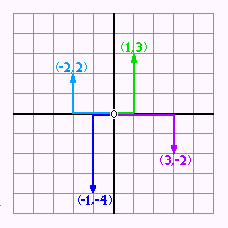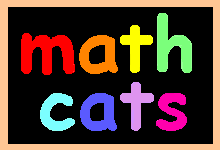Make Your Own Math Cats!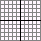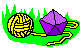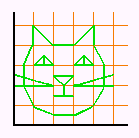You can use numbers to draw your own math cats! Here's how: Print out a grid and a chart with ordered pairs of numbers, such as (4,2). In each ordered pair, the first number tells you how far left or right to mark your point. The second number tells you how far up or down to mark your point. Plot each point on the grid. Draw a line from each point to the next to make a math cat.
 Math Cat #1 uses one quadrant. 0 is at the bottom left corner.To plot each point, first move to the right, then up.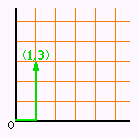Math Cat #2 uses 4 quadrants. 0 is in the center.To plot each point, first move right or left, then up or down.A negative number (such as -2) is less than 0. If the first number is negative, move to the left of 0. If the second number is negative, move below 0. You'll also need this 4 quadrant grid.